• 展开全部Dijkstra(迪杰斯特拉)算法是典型的最短路径路由算法，用于计算一个节点到其他所有节e68a8462616964757a686964616f31333361316131最短路径。主要特点是以起始为中心向外层层扩展，直到扩展到终点为止。...

展开全部

Dijkstra(迪杰斯特拉)算法是典型的最短路径路由算法，用于计算一个节点到其他所有节e68a8462616964757a686964616f31333361316131点的最短路径。主要特点是以起始点为中心向外层层扩展，直到扩展到终点为止。

Dijkstra一般的表述通常有两种方式，一种用永久和临时标号方式，一种是用OPEN, CLOSE表方式

用OPEN,CLOSE表的方式，其采用的是贪心法的算法策略，大概过程如下：

1.声明两个集合，open和close，open用于存储未遍历的节点，close用来存储已遍历的节点

2.初始阶段，将初始节点放入close，其他所有节点放入open

3.以初始节点为中心向外一层层遍历，获取离指定节点最近的子节点放入close并从新计算路径，直至close包含所有子节点

代码实例如下：

Node对象用于封装节点信息，包括名字和子节点

[java] view plain copy

public class Node {

private String name;

private Map child=new HashMap();

public Node(String name){

this.name=name;

}

public String getName() {

return name;

}

public void setName(String name) {

this.name = name;

}

public Map getChild() {

return child;

}

public void setChild(Map child) {

this.child = child;

}

}

MapBuilder用于初始化数据源，返回图的起始节点

[java] view plain copy

public class MapBuilder {

public Node build(Set open, Set close){

Node nodeA=new Node("A");

Node nodeB=new Node("B");

Node nodeC=new Node("C");

Node nodeD=new Node("D");

Node nodeE=new Node("E");

Node nodeF=new Node("F");

Node nodeG=new Node("G");

Node nodeH=new Node("H");

nodeA.getChild().put(nodeB, 1);

nodeA.getChild().put(nodeC, 1);

nodeA.getChild().put(nodeD, 4);

nodeA.getChild().put(nodeG, 5);

nodeA.getChild().put(nodeF, 2);

nodeB.getChild().put(nodeA, 1);

nodeB.getChild().put(nodeF, 2);

nodeB.getChild().put(nodeH, 4);

nodeC.getChild().put(nodeA, 1);

nodeC.getChild().put(nodeG, 3);

nodeD.getChild().put(nodeA, 4);

nodeD.getChild().put(nodeE, 1);

nodeE.getChild().put(nodeD, 1);

nodeE.getChild().put(nodeF, 1);

nodeF.getChild().put(nodeE, 1);

nodeF.getChild().put(nodeB, 2);

nodeF.getChild().put(nodeA, 2);

nodeG.getChild().put(nodeC, 3);

nodeG.getChild().put(nodeA, 5);

nodeG.getChild().put(nodeH, 1);

nodeH.getChild().put(nodeB, 4);

nodeH.getChild().put(nodeG, 1);

return nodeA;

}

}

图的结构如下图所示：

Dijkstra对象用于计算起始节点到所有其他节点的最短路径

[java] view plain copy

public class Dijkstra {

Set open=new HashSet();

Set close=new HashSet();

Map path=new HashMap();//封装路径距离

Map pathInfo=new HashMap();//封装路径信息

public Node init(){

//初始路径,因没有A->E这条路径,所以path(E)设置为Integer.MAX_VALUE

path.put("B", 1);

pathInfo.put("B", "A->B");

path.put("C", 1);

pathInfo.put("C", "A->C");

path.put("D", 4);

pathInfo.put("D", "A->D");

path.put("E", Integer.MAX_VALUE);

pathInfo.put("E", "A");

path.put("F", 2);

pathInfo.put("F", "A->F");

path.put("G", 5);

pathInfo.put("G", "A->G");

path.put("H", Integer.MAX_VALUE);

pathInfo.put("H", "A");

//将初始节点放入close,其他节点放入open

Node start=new MapBuilder().build(open,close);

return start;

}

public void computePath(Node start){

Node nearest=getShortestPath(start);//取距离start节点最近的子节点,放入close

if(nearest==null){

return;

}

open.remove(nearest);

Map childs=nearest.getChild();

for(Node child:childs.keySet()){

if(open.contains(child)){//如果子节点在open中

Integer newCompute=path.get(nearest.getName())+childs.get(child);

if(path.get(child.getName())>newCompute){//之前设置的距离大于新计算出来的距离

path.put(child.getName(), newCompute);

pathInfo.put(child.getName(), pathInfo.get(nearest.getName())+"->"+child.getName());

}

}

}

computePath(start);//重复执行自己,确保所有子节点被遍历

computePath(nearest);//向外一层层递归,直至所有顶点被遍历

}

public void printPathInfo(){

Set> pathInfos=pathInfo.entrySet();

for(Map.Entry pathInfo:pathInfos){

System.out.println(pathInfo.getKey()+":"+pathInfo.getValue());

}

}

/**

* 获取与node最近的子节点

*/

private Node getShortestPath(Node node){

Node res=null;

int minDis=Integer.MAX_VALUE;

Map childs=node.getChild();

for(Node child:childs.keySet()){

if(open.contains(child)){

int distance=childs.get(child);

if(distance

minDis=distance;

res=child;

}

}

}

return res;

}

}

Main用于测试Dijkstra对象

[java] view plain copy

public class Main {

public static void main(String[] args) {

Dijkstra test=new Dijkstra();

Node start=test.init();

test.computePath(start);

test.printPathInfo();

}

}

本回答由提问者推荐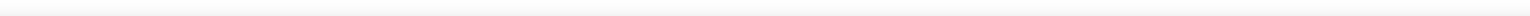已赞过

已踩过<

你对这个回答的评价是？

评论

收起

展开全文• 2.求两点间的最短路径（dijstra算法） 3.求两点间的最短路径（flyod算法） #include <iostream> #include <stdio.h> using namespace std; #define MAXVEX 100 #define INFI 0x3f3f3f typedef int ...

图是数据结构中很重要的一部分内容，这里我给出了在图的相关应用中最常见的几个算法实现。
1.dfs搜索所有节点。
2.求两点间的最短路径（dijstra算法）
3.求两点间的最短路径（flyod算法）

#include <iostream>
#include <stdio.h>
using namespace std;
#define MAXVEX 100
#define INFI 0x3f3f3f
typedef int VexType;
typedef int EdgType;
int visited[MAXVEX];
typedef struct
{ VexType Vexs[MAXVEX];
EdgType arc[MAXVEX][MAXVEX];
int vexnum,edgnum;
}MGraph;
void Create_Graph(MGraph &G)
{
int i,j,k,w;
cout<<"输入图的顶点数和边数"<<endl;
cin>>G.vexnum>>G.edgnum;
for(i=1;i<=G.vexnum;i++)
G.Vexs[i]=i;
for(i=1;i<=G.vexnum;i++)
for(j=1;j<=G.vexnum;j++)
G.arc[i][j]=INFI;
cout<<"输入顶点i,j,及它们间的权值："<<endl;
for(k=1;k<=G.edgnum;k++)
{

cin>>i>>j>>w;
G.arc[i][j]=w;
G.arc[j][i]=w;
}

}
void dfs(MGraph G,int i)
{ int j;
visited[i]=1;
cout<<G.Vexs[i]<<endl;
for(j=1;j<=G.vexnum;j++)
if(G.arc[i][j]<INFI&&!visited[j])
dfs(G,j);
}
void dijkstra(MGraph G) //迪杰斯特拉求单源点最短路径
{ int x;
printf("输入起点：");
cin>>x;
int book[MAXVEX],D[MAXVEX],P[MAXVEX],i,j,k,min;
for(i=1;i<=G.vexnum;i++)
{ book[i]=0;	//全部顶点初始化为未标记
D[i]=G.arc[x][i]; //将与x有顶点的连线加上权值
P[i]=x;          //初始化路径数组P为当前点下标
}
D[x]=0;//这个点到它本身的距离为0
book[x]=1; //初始化标记
for(i=1;i<=G.vexnum;i++)
{ min=INFI;
for(j=1;j<=G.vexnum;j++)  //寻找当前离v1最近的点
{ if(!book[j]&&min>D[j])
{ min=D[j];  //记录值
k=j;  //记录下标
}
}
book[k]=1; //标记这个点已走过
for(j=1;j<=G.vexnum;j++)
{
if(!book[j]&&min+G.arc[k][j]<D[j]) //如果经过k的顶点比当前这条路径长度短的话
{ D[j]=min+G.arc[k][j];
P[j]=k;
}
}
}
int des;
printf("输入终点:");
cin>>des;
printf("从%d到%d最短路径长度为:%d\n",x,des,D[des]);
printf("最短路径为：\n");
int a[MAXVEX],t=1;
a=des;
while(des!=x)//因为P数组存储着每个点的前驱，因此存到a数组里再逆序输出
{
a[t++]=P[des];
des=P[des];

}
printf("%d",a[t-1]);
for(i=t-2;i>=0;i--)
printf("->%d",a[i]);
printf("\n");
}
void Floyd(MGraph G) //弗朗依德求两点间最短路径
{ int v,w,k;
int D[MAXVEX][MAXVEX]; //存储最短路径长度
int P[MAXVEX][MAXVEX]; //存储最短路径
for(v=1;v<=G.vexnum;v++)
for(w=1;w<=G.vexnum;w++)
{ D[v][w]=G.arc[v][w];
P[v][w]=w;
}
for(k=1;k<=G.vexnum;k++)
for(v=1;v<=G.vexnum;v++)
for(w=1;w<=G.vexnum;w++)
{ if(D[v][w]>D[v][k]+D[k][w])
{ D[v][w]=D[v][k]+D[k][w];
P[v][w]=P[v][k];
}
}
cout<<endl;
cout<<"路径矩阵为："<<endl;
for(int i=1;i<=G.vexnum;i++)  //打印最短路径矩阵
{for(int j=1;j<=G.vexnum;j++)
cout<<P[i][j]<<" ";
cout<<endl;
}
for(int i=1;i<=G.vexnum;i++)  //打印任意两点最短路径
{for(int j=1;j<=G.vexnum;j++)
{if(i==j) continue;
cout<<i<<"->"<<j<<"的最短距离为:"<<D[i][j]<<endl;
cout<<"最短路径为："<<i;
k=P[i][j];
while(k!=j)
{ cout<<"->"<<k;
k=P[k][j];
}
cout<<"->"<<j<<endl;
}
}
/*cout<<"输入起点顶点:"<<endl;
cin>>source;
cout<<"输入终点顶点:"<<endl;
cin>>destination;
cout<<source<<"->"<<destination<<"最短路径为:"<<D[source][destination]<<endl;
cout<<source;*
k=P[source][destination];
while(k!=destination)
{ cout<<"->"<<k;
k=P[k][destination];
}
cout<<"->"<<destination<<endl;*/
}

{ printf("\n	--------1.深搜每个顶点-------");
printf("\n	--------2.floyd算法求最短路-------");
printf("\n	--------3.dijkstra算法求最短路-------\n");

}
int main()
{ MGraph G;
int source,destination,choice;
Create_Graph(G);

while(1)
{ printf("请输入您的选项：");
cin>>choice;
switch(choice)
{ 	case 1: cout<<"深度优先搜索为："<<endl; dfs(G,1);break;
case 2: Floyd(G);break;
case 3: dijkstra(G);break;
}

}

return 0;
}

运行结果如下：展开全文算法 数据结构 dfs dijkstra
• 最短路径-任意两点间最短距离-Floyd算法的matlab实现（详细教程） 目录 简介 核心思路 优缺点分析 算法过程 简介 Floyd算法又称为插点法，是一种利用动态规划的思想寻找给定的加权图中多源点之间最短路径的...

目录

简介

核心思路

优缺点分析

算法过程

简介

Floyd算法又称为插点法，是一种利用动态规划的思想寻找给定的加权图中多源点之间最短路径的算法，与Dijkstra算法类似。该算法名称以创始人之一、1978年图灵奖获得者、斯坦福大学计算机科学系教授罗伯特·弗洛伊德命名。

核心思路

路径矩阵

通过一个图的权值矩阵求出它的每两点间的最短路径矩阵。 

从图的带权邻接矩阵A=[a(i,j)] n×n开始，递归地进行n次更新，即由矩阵D(0)=A，按一个公式，构造出矩阵D(1)；又用同样地公式由D(1)构造出D(2)；……；最后又用同样的公式由D(n-1)构造出矩阵D(n)。矩阵D(n)的i行j列元素便是i号顶点到j号顶点的最短路径长度，称D(n)为图的距离矩阵，同时还可引入一个后继节点矩阵path来记录两点间的最短路径。

采用松弛技术（松弛操作），对在i和j之间的所有其他点进行一次松弛。所以时间复杂度为O(n^3);

状态转移方程

状态转移方程如下： map[i,j]:=min{map[i,k]+map[k,j],map[i,j]}；

map[i,j]表示i到j的最短距离，K是穷举i,j的断点，map[n,n]初值应该为0，或者按照题目意思来做。

当然，如果这条路没有通的话，还必须特殊处理，比如没有map[i,k]这条路。

优缺点分析

编辑 语音

Floyd算法适用于APSP(All Pairs Shortest Paths，多源最短路径)，是一种动态规划算法，稠密图效果最佳，边权可正可负。此算法简单有效，由于三重循环结构紧凑，对于稠密图，效率要高于执行|V|次Dijkstra算法，也要高于执行|V|次SPFA算法

优点：容易理解，可以算出任意两个节点之间的最短距离，代码编写简单。

缺点：时间复杂度比较高，不适合计算大量数据

算法过程

在Floyd算中一般会用到有两个矩阵，一个距离矩阵D一个路由矩阵R，顾名思义距离矩阵D是用来储存任意两点之间的距离的，而路由矩阵则是用来记录任意两点之间的路径关系。

Floyd算法的原理是：对于每一对顶点 i 和 j，看看是否存在一个顶点 w 使得从 i 到 w 再到 j 比已知的路径更短。如果是更新它。

把图用邻接矩阵r表示出来，如果从Vi到Vj有路可达，则D[i，j]=d(在矩阵D中为具体的数字)，d表示该路的长度；否则D[i，j]=无穷大（在矩阵D中用“inf“表示）。定义一个矩阵D用来记录所插入点的信息，R[I,j]表示从Vi到Vj需要经过的点，初始化R[i,j]=j（即先默认i到j是通的）。把各个顶点插入图中，比较插点后的距离与原来的距离，假设插入的中间点为k，D[i,j] > D[i,k]+D[k,j] )，此时证明从i点经过k点再到j点比原来的要短，所以此时要更新D[i,j],则D[i,j]=D[I,k]+[k,j],同时此时R[i,j]=k。在R中包含有两点之间最短道路的信息，而在D中则包含了最短通路径的信息。

可能有些人对路由矩阵R不是很明白，其实路由矩阵R很好理解，我来举个例子，

比如，要寻找从V5到V1的路径。根据R，假如 R(5,1)=3则说明从V5到V1经过V3，路径为{V5,V3,V1}，如果R(5,3)=3，说明V5与V3直接相连，如果R(3,1)=1，说明V3与V1直接相连。

因此，我们在定义路由矩阵R时，先要初始化矩阵（即先默认任意两点是相互相通的），即每列上的数等于该列的列序数。

例：

 V1 V2 V3 V4 **** Vn V1 1 2 3 4 **** n V2 1 2 3 4 **** n V3 1 2 3 4 **** n V4 1 2 3 4 **** n **** 1 2 3 4 **** n Vn 1 2 3 4 **** n

示例

下面我将用一个简单的例子来说明，下面是一个简单的例子：

问题：求出任意两点间的最短距离及其路径？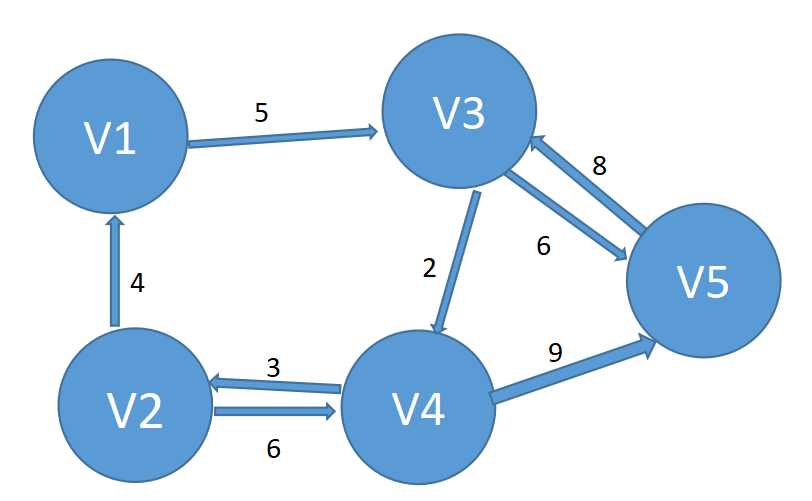我们此时可以写出距离矩阵D和路由矩阵R如下：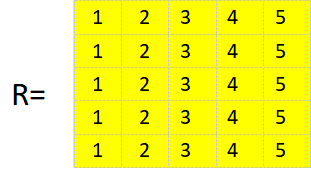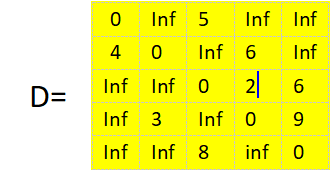这样我们就定义好了距离矩阵路由矩阵，现在我们再来假设图中任意两点之间都要经V5这个点中转。算出经过中转后的两点之间的距离，然后我们再来判断任意两点之间的最短距离。
（1）先从V5开始，先让V5成为中转点。

V5由V5中转，那么V5到到各个点的距离还是不变。

V4可以经由V5中转，那么这个时候判断一下中转前和中转后的距离大小，将最小距离留存下来如：

V4>V3 经V5中转，原来V4->V3 = inf，经由V5中转之后V4->V5->V3 = 17, 于是V4到V3的最短距离变化为17，更新距离矩阵D(4,3)=17,更新路由矩阵R(4,3) = R(4,5) = 5

V1、V2、V3没有到达V5的路径，所以也就不存在从V5中转的情况，所以V1、V2、V3到各个点的距离还是不变。

这时两个矩阵变化为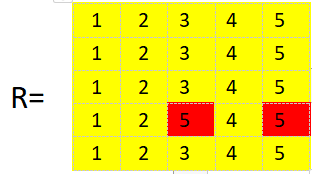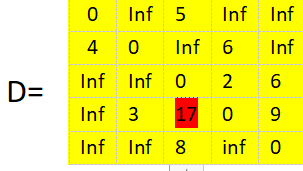（2）再让V4成为中转点。

V5  V1 都没有到达V4的路径，所以也就不存在从V5中转的情况，所以V5 V1 到各个点的距离还是不变。

V2>V5 经V4中转，原来V2->V5 = inf，经由V4中转之后V2->V4->V5 = 15, 于是V2到V5的最短距离变化为15，更新距离矩阵D(2,5)=15,更新路由矩阵R(2,5) = R(2,4) = 4

V3>V2经V4中转，原来V3->V2= inf，经由V4中转之后V3->V4->V2 = 5, 于是V3到V2的最短距离变化为5，更新距离矩阵D(3,2)=5,更新路由矩阵R(3,2) = R(3,4) = 4

V3>V5 经V4中转，原来V3->V5 = 6，经由V4中转之后V3>V4->V5 = 11, 因此V3到V5的最短距离仍为6，不更新。

这时两个矩阵变化为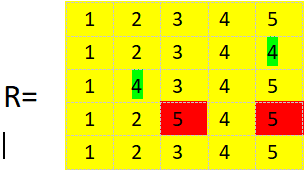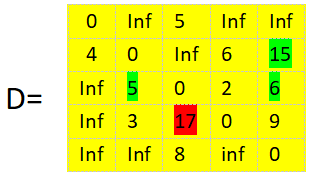(3)同理，让所有的点都成为一次中转点

用Matlab来表示上面的过程，其代码为：

（1）先建立一个.m文件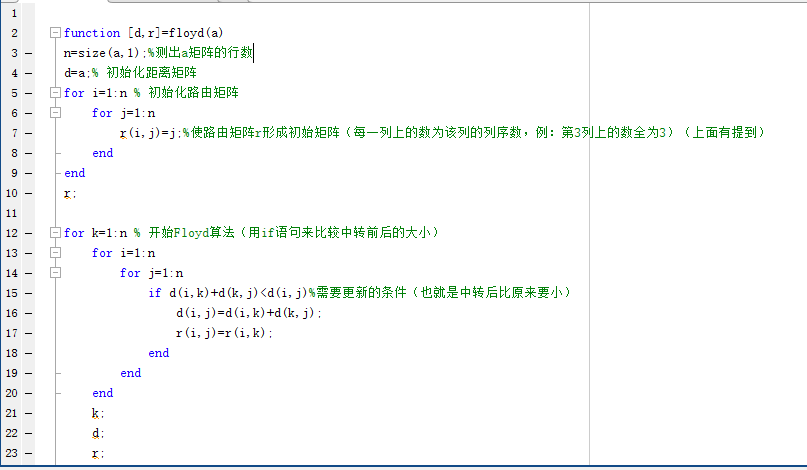function [d,r]=floyd(a)
n=size(a,1);%测出a矩阵的行数
d=a;% 初始化距离矩阵
for i=1:n % 初始化路由矩阵
for j=1:n
r(i,j)=j;%使路由矩阵r形成初始矩阵（每一列上的数为该列的列序数，例：第3列上的数全为3）（上面有提到）
end
end
r;

for k=1:n % 开始Floyd算法（用if语句来比较中转前后的大小）
for i=1:n
for j=1:n
if d(i,k)+d(k,j)<d(i,j)%需要更新的条件（也就是中转后比原来要小）
d(i,j)=d(i,k)+d(k,j);
r(i,j)=r(i,k);
end
end
end
k;
d;
r;

(2)在命令行输入矩阵a，也就是距离矩阵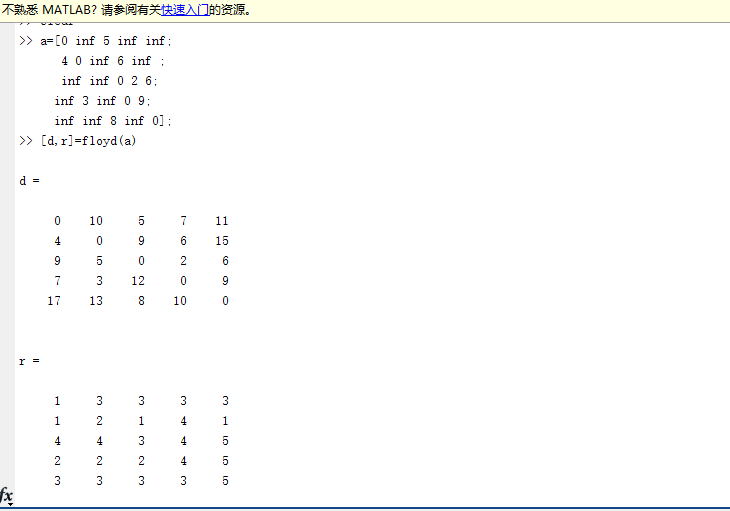> a=[0 inf 5 inf inf;
4 0 inf 6 inf ;
inf inf 0 2 6;
inf 3 inf 0 9;
inf inf 8 inf 0];
>> [d,r]=floyd(a)

（3）得出距离矩阵D和路由矩阵R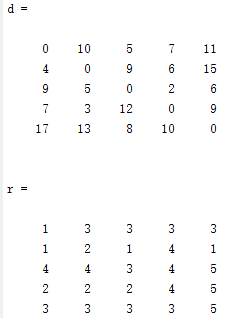（4）怎么通过得出的这两个矩阵看任意一点到任意一点的最短距离及其路径。

从任意一点到任意一点从矩阵d中就很容易看出来了，比如D(2,3)=9,就表示V2到V3的最短距离是9，因此两点的距离从矩阵中很容易看出，我就不过多解释了。

现在我重点来说一下怎么看路由矩阵：

举个例子：v4->V3

从距离矩阵中可以看出V4->V3的最短距离是D(4,3) = 12；根据其路由矩阵我们可以看出：

R(4,3) = 2,表示V4->V3,先经过V2，于是再看R(2,3) = 1,表示还需要再经过V1,于是我们看R(1,3) = 3,这个时候我们发现终于到了V3,所以我们梳理一下，V4->V3的最短路径是：V4->V2->V1->V3。简言之就是固定列，根据路由矩阵在行中跳转，直到跳转到对应的点。

再来个例子：v5->V2

从距离矩阵中可以看出V5->V2的最短距离是D(5,2) = 13；根据其路由矩阵我们可以看出：

R(5,2) = 3,表示V5->V2,先经过V3，于是再看R(3,2) = 4,表示还需要再经过V4,于是我们看R(4,2) = 2,这个时候我们发现终于到了V2,所以V5->V2的最短路径是：V4->V2->V1->V3。

此时我们已经拿到了我们最开始想要的结果了！！！

展开全文matlab 算法 动态规划
• importjava.util.Scanner;//最短路径求解public classDistMin {static classGraphMatrix{static final int MaxNum=20;char[] Vertex=new char[MaxNum]; //保存顶点信息(序号或字母)int GType; //图的类型(0：...

packagecom.cn.datastruct;importjava.util.Scanner;//最短路径求解

public classDistMin {static classGraphMatrix{static final int MaxNum=20;char[] Vertex=new char[MaxNum]; //保存顶点信息(序号或字母)

int GType; //图的类型(0：无向图，1：有向图)

int VertexNum; //顶点的数量

int EdgeNum; //边的数量

int[][] EdgeWeight=new int[MaxNum][MaxNum]; //保存边的权

int[] isTrav=new int[MaxNum]; //遍历标志

}static final int MaxValue=65535; //最大值(可设为一个最大整数)

static int[] path=new int[GraphMatrix.MaxNum]; //两点经过的顶点集合的数组

static int[] tmpvertex=new int[GraphMatrix.MaxNum]; //最短路径的起始点集合

static Scanner input=newScanner(System.in);//创建邻接矩阵图

static voidCreateGraph(GraphMatrix GM){inti,j,k;int weight; //权

char EstartV,EendV; //边的起始顶点

System.out.printf("输入图中各顶点信息\n");for(i=0;i

System.out.printf("第%d个顶点：", i+1);

GM.Vertex[i]=(input.next().toCharArray()); //保存到各顶点的数组元素中

}

System.out.printf("输入构成各边的顶点及权值：\n");for(k=0;k

System.out.printf("第%d条边：", k+1);

EstartV=input.next().charAt(0);

EendV=input.next().charAt(0);

weight=input.nextInt();for(i=0;EstartV!=GM.Vertex[i];i++); //在已有顶点中查找始点

for(j=0;EendV!=GM.Vertex[j];j++); //在已有的顶点中查找终点

GM.EdgeWeight[i][j]=weight; //对应位置保存权值，表示有一条边

if(GM.GType==0){ //若是无向图

GM.EdgeWeight[j][i]=weight; //在对角位置保存权值

}

}

}//清空矩阵

static voidClearGraph(GraphMatrix GM) {inti, j;for (i = 0; i < GM.VertexNum; i++) {for (j = 0; j < GM.VertexNum; j++) {

GM.EdgeWeight[i][j]= MaxValue; //设置矩阵中各元素的值为MaxValue

}

}

}//输出邻接矩阵

static voidOutGraph(GraphMatrix GM) {inti, j;for (j = 0; j < GM.VertexNum; j++) {

System.out.printf("\t%c", GM.Vertex[j]); //在第一行输出顶点信息

}

System.out.println();for (i = 0; i < GM.VertexNum; i++) {

System.out.printf("%c", GM.Vertex[i]);for (j = 0; j < GM.VertexNum; j++) {if (GM.EdgeWeight[i][j] == MaxValue) { //若权值为最大值

System.out.printf("\tZ"); //以Z表示无穷大

} else{

System.out.printf("\t%d", GM.EdgeWeight[i][j]); //输出边的权值

}

}

System.out.println();

}

}//最短路径算法

static void distMin(GraphMatrix GM,int vend){ //vend为结束点

int[] weight=new int[GraphMatrix.MaxNum]; //某终止点到各顶点的最短路径长度

inti,j,k,min;

vend--;for(i=0;i

weight[i]=GM.EdgeWeight[vend][i];

}for(i=0;i

if(weight[i]0){ //有效权值

path[i]=vend;

}

}for(i=0;i

tmpvertex[i]=0; //初始化顶点集合为空

}

tmpvertex[vend]=1; //选入顶点vend

weight[vend]=0;for(i=0;i

min=MaxValue;

k=vend;for(j=0;j

min=weight[j];

k=j;

}

}

tmpvertex[k]=1; //将顶点k选入

for(j=0;j

if(tmpvertex[j]==0&&weight[k]+GM.EdgeWeight[k][j]

weight[j]=weight[k]+GM.EdgeWeight[k][j];

path[j]=k;

}

}

}

}public static voidmain(String[] args) {

GraphMatrix GM=new GraphMatrix(); //定义保存邻接表结构的图

String go;intvend;inti,k;

System.out.println("求解最短路径问题！");do{

System.out.print("请先输入生成图的类型：");

GM.GType=input.nextInt(); //图的种类

System.out.print("请输入图的顶点数量：");

GM.VertexNum=input.nextInt(); //输入图中顶点数

System.out.print("请输入图的边的数量：");

GM.EdgeNum=input.nextInt(); //输入图中边数

ClearGraph(GM); //清空图

CreateGraph(GM); //生成邻接表结构的图

System.out.print("\n请输入结束点：");

vend=input.nextInt();

distMin(GM,vend);

vend--;

System.out.printf("\n个顶点到达顶点%c的最短路径分别为(起始点-结束点)：\n",GM.Vertex[vend]);for(i=0;i

if(tmpvertex[i]==1){

k=i;while(k!=vend){

System.out.printf("顶点%c-", GM.Vertex[k]);

k=path[k];

}

System.out.printf("顶点%c\n", GM.Vertex[k]);

}else{

System.out.printf("%c-%c:无路径\n", GM.Vertex[i],GM.Vertex[vend]);

}

}

System.out.println("\n继续玩吗(y/n)?");

go=input.next();

}while(go.equalsIgnoreCase("y"));

System.out.println("游戏结束！");

}

}

展开全文• floyd算法：计算任意两点之间的最短路径 基于动态规划思想 三重循环遍历所有顶点，如果a到c再到b的距离，小于a到b的距离，那么将a到b原来的路径更换为a到c再到b 其中路径信息parent矩阵，parent[i][j]代表的是，从i...
• title: 图的最短路径算法 date: 2019-02-12 19:33:29 文章目录0. 前言1. 迪杰斯特拉 Dijkstra2....迪杰斯特拉算法，用于解决 “给定起始到其余的最短路径” 问题，即单源最短路径算法。时间复杂度为 O(n.算法
• 2、循环n - 1 次，每次选出一个距离最小的，将其状态更新为visited，然后更新其他所有与起点距离 #include <iostream> #include <cstring> using namespace std; const int N = 510, INF = 0x3f3...算法 数据结构
• 任务描述：在一个无向图中，获取起始节点到所有其他节点的最短路径描述Dijkstra(迪杰斯特拉)算法是典型的最短路径路由算法，用于计算一个节点到其他所有节点的最短路径。主要特点是以起始为中心向外层层扩展，直到...java最短路径算法
• 使用字典的方式构建有向图，并搜索图中的路径。 提供了三种不同的操作函数： 不足：只能够处理无权无向图 #这个图有6个节点(A-G)和8个弧。它可以通过下面的Python数据结构来表示： graph = {'A': ['B', 'C','D'], ...算法 python 数据结构
• 关于最短距离算法最多莫...书上讲的Dijkstra算法看的不太懂，大致的意思是扩散求值的算法，即从源节点一步一步求最短路径的方法。即先找到源节点到其子节点的最短距离，然后再找源节点到其二级孙级最短距离。如此...
• Bellman-ford算法、SPFA算法和 Dijkstra算法在处理单源最短路径问题时候的异同。单源最短路径 Dijkstra SPFA 最短路径
• 这是使用BFS从(0,0)到(0，m-1)的矩阵中的最短路径的python实现 . 您可以将其更改为适合变量 .n,m,k1,k2=[int(i) for i in input().split()]arr=[[int(j) for j in input().split()] for i in range(n)]x=[[-1 for ...
• #include //假设取10个节点，N=28//定义无穷大为100000#define N ...//最短路径int path[N];//上一节点int is[N];//是否选用该节点,0位未选用double destination[N][N]={{0, 97.9, 100000, 100000, 227.2, 100000, ...
• #include#include#include#define INFINITY 32768 //无穷大#define MAX_VERTEX_NUM 11 //最大顶点数typedef struct... //路径长度，对于无权图0-1-表示是否相邻，对于带权图则为权值}ArCell;typedef struct //图中顶...
• 求K条最短路径的必要性最短路径问题分为：单源最短路径所有顶点对间的最短路径共同的缺陷：这里的最短路径两点间最短的那一条路径，不包括次短、再次短等路径。这样的最短路径问题比较狭义。在实际情况中，例如：...
• 目录前言一、Dijkstra算法算法实现二、Floyd-Warshall 算法算法实现 前言 ...在图问题中，这一问题对应的算法被称为最短路径算法。本文介绍其中种非常著名算法的JavaScript实现： Dijkstra算法和F算法 javascript 开发语言
• Floyd算法:思路 ：遍历计算 i 经过 k 到 j 的最小路径值 (动态规划思路)缺点：时间复杂度高，不能解决负边情况输入样例：4 81 2 21 3 61 4 42 3 33 1 73 4 14 1 54 3 12输出样例：1-->2:21-->3:51--&...
• 多节点最短路径算法——改进型dijstra（迪杰斯特拉）算法 原dijstra为单节点最短路径算法，这里是做了一些改进，通过前面已知各个节点间的权重，计算出两两节点间的最短路径。 clc,clear all a=zeros(6); a(1,2)=50;...算法 matlab
• 迪杰斯特拉算法介绍迪杰斯特拉(Dijkstra)算法是典型最短路径算法，用于计算一个节点到其他节点的最短路径。它的主要特点是以起始为中心向外层层扩展(广度优先搜索思想)，直到扩展到终点为止。基本思想通过Dijkstra...
• 包括的经典的Dijkstra算法，Floyd算法和Bellman-Ford 算法
• 最短路径算法

2021-01-03 01:01:44
最短路径算法：Dijkstra和Bellman Dijkstra 问题：在一张图中，从一点出发到大其他个的最短路径。 思路：假设a到b之前的直达路径是10，如果求a到b的最短路径，就需要抄近路，比如加个c，a->c->b，看这...算法
• /**** 带权重有方向图* 介绍了Dijkstra算法：最短路径算法** Dijkstra算法原理：* 1) 适用条件&范围：a) 单源最短路径(从源点s到其它所有顶点v);b) 有向图&无向图(无向图可以看作(u,v),(v,u)同属于边集E的有...
• Dijkstra算法类似于贪心算法计算从起点到指定顶点的最短路径，通过不断选择距离起点最近的顶点，来逐渐扩大最短路径权值，直到覆盖图中所有顶点（与方法4不同，其对边的松弛顺序是随意进行的）。其应用根本在于最短...python 算法 数据结构
• 介绍迪杰斯特拉(Dijkstra)算法是典型的最短路径算法，用于计算一个结点到其他结点的最短路径。它的主要特点是以起始为中心向外层层扩展(广度优先搜索思想)，直到扩展到终点为止。基本思想通过Dijkstra计算图G中的...
• 本文总结了图的几种最短路径算法的实现：深度或广度优先搜索算法，费罗伊德算法，迪杰斯特拉算法，Bellman-Ford 算法。 1）深度或广度优先搜索算法（解决单源最短路径） 从起点开始访问所有深度遍历路径或广度优先...算法 数据结构 深度学习
• 目录一、算法分析二、代码实现三、测试... 顶点i，j间的距离定义为从i出发到j的最短路径长度。目的：找出图G中每一个顶点到其他所有顶点的距离(有向图，即A到B与B到A的距离可能不一样)。约定：若(i,j)∉E，则w[i,...
• 算法(二) 最短路经 Shortest Path Dijkstra算法练习题链接 /vjudge/contest/view.action?cid=29337#overview Floyd算法练习题链接 /vjudge/contest/view.action?cid=29305#overview 密码都是：671 /sjjg/...数据结构最短路径例题
• Dijkstra也叫迪杰斯特拉，是典型最短路径算法，计算一个起始节点到路径中其他所有节点的最短路径的算法和思想。在一些专业课程中如数据结构，图论，运筹学等都有介绍。其思想是一种基础的求最短路径的算法，通过基础...
• 前言本文同步于公众号[bigsai]，专注于数据结构与算法、java、python在图论中，在寻路最短路径中除了Dijkstra算法以外，还有Floyd算法也是非常经典，然而算法还是有区别的，Floyd主要计算多源最短路径。...JAVA弗洛伊德算法...